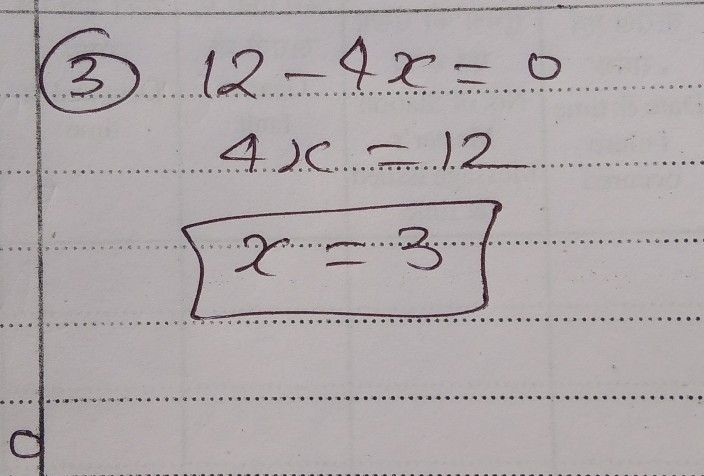Symbol
Problem$1.$ $3m+8=15$ $2.$ $x^{2}-5x+10=0$ $3.$ $12-4x=0$ $4.$ $2t^{2}$ $-7t=12$ $5$ $6-2x+3x^{2}=0$ $6$ $25-r^{2}=4x-5x^{2}$
7th-9th grade
Other
Search count: 105
SolutionQanda teacher - Nirmalya